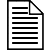# Planning Introductions

Now that you have a loose outline of your lesson, think about how you'll introduce it. Introductions are important because, like transitions, they guide students' understanding of the course and its goals. When you provide an introduction, students see that you have a sense of where the lesson is headed. Not only will this add to your credibility, but students will be less inclined to ask, "Why do we have to do this?"

Use introductions to connect concepts from earlier classes to the upcoming lesson. Also use them as checkpoints or reminders for yourself and your students - this is where we've been and this is where we're going.

When writing introductions, look back at the previous lesson and tie up any loose ends. Perhaps students were walking out the door when you explained the connection between an activity and an upcoming essay assignment. Introductions are ideal times to reinforce important concepts.More on Introductions